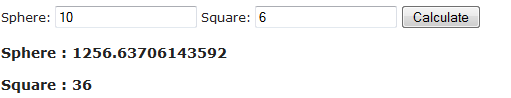### Virtual modifier in C#. Practical example in Asp.Net MVC

In this tutorial I will focus on creating virtual method that we can override.
I will use ASP.NET MVC as my main framework to display the results.
Methods are by default non virtual, to enable override you have to add virtual keyword.

Virtual modifier cannot be used with : static, override, sealed.

We will create a base class Dimension and 2 classes that inherit from it Square and Sphere.

Model

public class Shapes
{
public class Dimension
{
public const double PI = Math.PI;
protected double x, y;

public Dimension()
{

}

public Dimension(double x, double y)
{
this.x = x;
this.y = y;
}

public virtual double Area()
{
return x * y;
}
}

public class Square : Dimension
{
public Square(double side) : base(side,0)
{

}

public override double Area()
{
return x*x ;
}

}

public class Sphere : Dimension
{
public Sphere(double r)
: base(r, 0)
{
}

public override double Area()
{
return 4 * PI * x * x;
}
}

}

// To get the values from View that user entered
public class SelectedValue
{
public double sphere { get; set; }
public double square { get; set; }
}

We will make this application more interesting so rather hard code value, we give user ability to enter values for shapes.

View

@model SelectedValue

@using(Html.BeginForm("Shapes", "Home", FormMethod.Post))
{
<span>Sphere: </span>@Html.TextBoxFor(m=>m.sphere)
<span>Square: </span>@Html.TextBoxFor(m=>m.square)
<input type="submit" value= "Calculate" />
}

@if (ViewData["sphereArea"] != null)
{
<h3>Sphere : @ViewData["sphereArea"]</h3>
}

@if (ViewData["squareArea"] != null)
{
<h3>Square : @ViewData["squareArea"]</h3>
}

CONTROLLER

In controller method Shapes simply get the value from model that was passed from view and call the appropriate method in Shapes class. As you can see Area method is called in Sphere and Square, but return different value. This is accomplished by using virtual keyword on our method and override keyword.

public ActionResult Index()
{

Shapes.Sphere sphere = new Shapes.Sphere(5);

ViewData["sphere"] = sphere.Area();

return View();
}

public ActionResult Shapes(SelectedValue model)
{
double SphereArea = new Shapes.Sphere(model.sphere).Area();
double SquareArea = new Shapes.Square(model.square).Area();

ViewData["sphereArea"] = SphereArea;
ViewData["squareArea"] = SquareArea;

return View("Index");
}

After following this tutorial you should achieve this results.1.1.